# Special Points

## Overview

There are certain points on the graph of any non-linear function where the curve makes a significant transition - or in some cases simply does not exist. All of these special points fall into one or more of the following categories:

• Maxima and minima
• Turning points
• Critical (or stationary) points
• Inflection points

Note that these categories are far from being mutually exclusive, as we shall see. At this point you might be wondering why we are interested in such points. There are in fact all sorts of reasons why they can be of interest to scientists, engineers, mathematicians and those working in commerce and industry. Solving optimisation problems is one of the most common examples. We often want to know what input value to use in order to achieve the maximum output from a function, for example when generating power or maximising profit. Conversely, we might want to find the correct input values to use in order to minimise something, like power consumption or material costs.

We can often see where these special points occur by plotting the graph of a function. Sometimes, however, we need to pinpoint their location more precisely. Although the techniques used to accomplish this are dealt with elsewhere, it is worth mentioning here that we can use differential calculus to identify the location of maxima and minima, turning points, critical points and inflection points. The derivative of a function at a turning point, for example, will always be zero. So, although not every point at which the derivative is zero will be a turning point, we can identify any points that might be turning points by finding all the points on a function graph that have a derivative of zero.

## Maxima, minima and turning points

We'll start by looking at examples of maxima and minima. A maximum or minimum point of a function is called an extremum. Maxima or minima are collectively called extrema. A point will often (though not always) be both an extremum and a turning point. Let's look at the graph of the continuous non-linear function ƒ(x) = 2x 3 - 3x 2 - 3x + 2:The graph of the non-linear function ƒ(x) = 2x 3 - 3x 2 - 3x + 2

The function ƒ(x) = 2x 3 - 3x 2 - 3x + 2 has three real roots (in other words, the graph intersects the x axis at three points). The function also has a local maximum and a local minimum, which we have labelled on the graph. The word local is used to indicate that we are talking about the maximum or minimum value of y for a limited range of values of x, rather than over the entire domain of the function (the domain of a function is the complete set of input values for which the function is defined). In other words, local extrema are points that have the largest or smallest y values relative to their immediate neighbours. Such extrema are also turning points. A turning point is a point at which the y coordinate ceases to increase in a particular direction, and begins to increase in the opposite direction.

At the local minimum shown on the graph, we can see that the value of y has stopped falling. Beyond this point, it starts to rise again as x increases. However, for negative values of x that are significantly less than minus one, the corresponding value of y will be less than this local minimum. Indeed, as the value of x approaches minus infinity (-∞), the value of y will also approach minus infinity. The same thing applies to the point indicated on the graph as a local maximum. Here, the value of y has stopped increasing, and starts to fall again as x increases. However, once x becomes significantly greater than two (2), the value of y becomes greater than the local maximum. It will continue to increase indefinitely as x increases, and as the value of x approaches infinity, the value of y will also approach infinity. Because the function extends to infinity in both the positive direction and the negative direction, it cannot have either a global maximum or a global minimum.

The global maximum, if it exists, is the largest value that y can have over the entire domain of the function. Similarly, the global minimum, if it exists, is the smallest value that y can have over the entire domain of the function. In some cases, a function may have both a global maximum and a global minimum. Obvious examples include the trigonometric sine and cosine functions, both of which are cyclic. Note that for these particular functions, the global maximum and all local maxima will all have exactly the same value. The same is true of the global minimum and local minima.The sine function has an infinite number of local extrema

The function ƒ(x) = -2x 2 + 2 is an example of a function that has a global maximum. The graph of the function is shown below. Note that the graph extends downwards to negative infinity on either side of the y axis. The function therefore has no global minimum. It also has no local minima, although it should be noted that the global maximum is also both a turning point and a local maximum. At this point we should perhaps clarify the difference between a global maximum and a local maximum. We saw above that in cyclic functions like the sine function, the global maximum occurs for an infinite number of different values of x, but it will always have the same value. A global maximum can only ever have a single value. This is not the case with local maxima. The sine function has an infinite number of local maxima that all take the same value. Other functions may have multiple local maxima, each of which can take a different value, as we shall see.The graph of the non-linear function ƒ(x) = -2x 2 + 2

The function ƒ(x) = x 2 - 1 is an example of a function that has a global minimum. The graph of the function is shown below. Note that this time the graph extends upwards to infinity on both sides of the y axis. The function therefore has no global maximum. It also has no local maxima, but the global minimum is also both a turning point and a local minimum. Like the global maximum, a global minimum can only ever have a single value. Multiple local minima within the same function, like multiple local maxima, can have different values.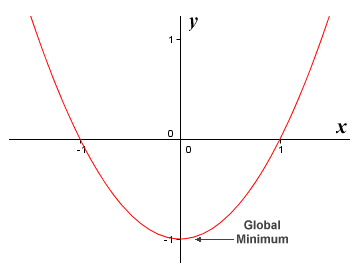The graph of the non-linear function ƒ(x) = x 2 - 1

The following illustration shows the graph of the function ƒ(x) = x + sin (2x). Note that for this function, there will be an infinite number of both local maxima and local minima, each of which will have a unique value. Because the values returned by the function continue to infinity in both the positive and the negative direction, however, there are no global extrema.The graph of the non-linear function ƒ(x) = x + sin (2x)

So far we have only really looked at extrema (maxima and minima) in continuous functions (i.e. functions that do not have gaps or sudden breaks). We have also not yet considered a scenario in which we are only interested in a function over a specific interval (i.e. a range of x values bounded by some finite minimum and maximum values of x). However, not all functions are continuous. It is also often the case that we are only interested in a function over some finite interval. If we do restrict the domain of the function in this way, there will always be a global maximum and a global minimum. The graph of the fifth order polynomial function ƒ(x) = x 5 + 3.5x 4 - 3x 3 - 13x 2 + x + 3 is shown below. We are only interested here in the interval between the x values minus three (-3) and one-point-five (1.5).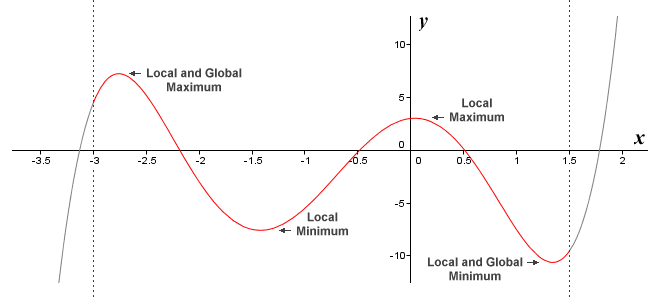The graph of the non-linear function ƒ(x) = x 5 + 3.5x 4 - 3x 3 - 13x 2 + x + 3

Note that because we have restricted the function to a finite interval, we must have a global maximum and a global minimum. In the above example, we have a total of four local extrema, because the global extrema also happen to be local extrema. Note also that all of these extrema are also turning points. It would of course be perfectly possible to define an interval on this function (or any function, for that matter) that contained no local extrema. It would still have global extrema, however, since there would always be some maximum and minimum point.

Note that by definition (we will be looking at some formal definitions in due course), the end point of a function cannot be a local extremum. It can, however, be a global extremum. We can demonstrate this last point by defining a different interval on the function ƒ(x) = x 5 + 3.5x 4 - 3x 3 - 13x 2 + x + 3. This time, we will restrict ourselves to the interval between the x values minus two-point-five (-2.5) and one-point-two-five (1.25).Choosing a different interval can change the global extrema

Now that we have redefined the interval in which we are interested, we have a new global maximum and a new global minimum. Although two of the local extrema we had previously are still in evidence, the new global extrema cannot be local extrema because they are both end points. An extremum can only be considered local if it falls within a defined interval. It cannot be an end point. A similar restriction applies to turning points. A turning point can only occur within a defined interval, and not at an end point.

Another possibility we should allow for is that a function may be discontinuous at some point. Consider the following split function (this type of function is sometimes called a piecewise-defined function):

 ƒ(x)  = { x 5 + 3.5x 4 - 3x 3 - 13x 2 + x + 3 for x ≤ -1 x 5 + 3.5x 4 - 3x 3 - 13x 2 + x - 3 for x > -1

What we are saying here is that, for any value of x up to and including minus one (-1), our original function of ƒ(x) = x 5 + 3.5x 4 - 3x 3 - 13x 2 + x + 3 will apply. For all values of x greater than minus one, the function ƒ(x) = x 5 + 3.5x 4 - 3x 3 - 13x 2 + x - 3 will apply instead. The difference is actually a fairly minor one. For values of x greater than minus one, the constant term in the function changes from three (3) to minus three (-3). The effect is to shift the graph downwards for values of x greater than minus one, creating a discontinuity. The graph of the split function is shown below. Note that we have also redefined the interval in which we are interested to include only x values falling between minus two-point-five (-2.5) and zero-point-five (0.5).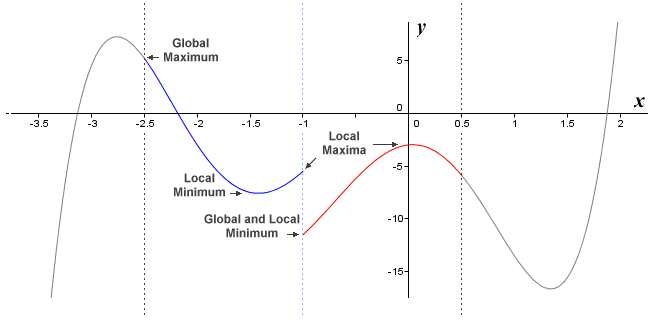Global and local extrema may occur at a discontinuity

We now have four local extrema, a global maximum that occurs at an endpoint, and a global minimum that occurs on a discontinuity (which is also one of the local minima). Note that a local extremum can occur at a discontinuity, even though one cannot occur at an end point. Although defining a finite interval guarantees that we will have both global extrema (providing the function is continuous), there is no such requirement for local extrema. It is perfectly possible for a function defined on an interval to have no local extrema. If the function is defined on a finite interval but has a discontinuity, the existence of global extrema rather depends on the type of discontinuity that occurs. If a function is not bounded by an interval, whether continuous or not, it is often the case that it will have either a single global extremum or no global extrema at all. In fact, an unbounded function may have no extrema of any kind.

Before we move on to consider critical points, here is a summary of the various types of extrema we have seen, together with some formal definitions:

• Local maximum – a function can be said to have a local maximum if there is some point within the domain of the function (excluding end points) that has a y value that is greater than that of any of its immediate neighbours. Stating this more formally, we say that ƒ(x) has a local maximum at x = c if ƒ(c) ≥ ƒ(x) for every x in some open interval around x = c.
• Local minimum – a function can be said to have a local minimum if there is some point within the domain of the function (excluding end points) that has a y value that is less than that of any of its immediate neighbours. Stating this more formally, we say that ƒ(x) has a local minimum at x = c if ƒ(c) ≤ ƒ(x) for every x in some open interval around x = c.
• Global maximum – a function can be said to have a global maximum if there is some point within the domain of the function that has a y value that is greater than that of any other point in the domain. Stating this more formally, we say that ƒ(x) has a global maximum at x = c if ƒ(c) ≥ ƒ(x) for every x in the domain.
• Global minimum – a function can be said to have a global minimum if there is some point within the domain of the function that has a y value that is less than that of any other point in the domain. Stating this more formally, we say that ƒ(x) has a global minimum at x = c if ƒ(c) ≤ ƒ(x) for every x in the domain.

The term "open interval around x = c" used in the above descriptions of local extrema refers to some interval bounded by the x values a and b for which we can say that a < c < b.

## Critical points

As you may remember, we stated earlier that the various categories of special points were not mutually exclusive. What we meant was that a special point could belong to more than one category simultaneously. All turning points, for example, are also critical points. They will also be local extrema, and may even be global extrema as well, but it is the characteristics of critical points that we are interested in here.

There are several kinds of critical point. One kind of critical point (sometimes called a stationary point) is a point on the graph of a function at which the derivative (i.e. the slope of the graph) is zero (0). The tangent to the graph at that point is parallel to the x axis. This kind of critical point will include all turning points. The other kinds of critical points share one thing in common, which is that at these points, the function has a value but the derivative does not exist. Stating all this formally, we can say that a function ƒ(x) has a critical point at x = c if ƒ(c) exists and either

 d ƒ(c)  =  0 dx

or

 d ƒ(c) does not exist dx

We have already seen examples of critical points when we looked at maxima and minima, because all extrema are critical points. Some extrema are critical points by virtue of the fact that they are turning points, and will therefore have a derivative of zero. Other extrema are critical points because they occur at a discontinuity, or because they occur at the end point of an interval. In either case, the derivative will not exist. A few examples would probably be helpful at this point.

Here is the graph of the function ƒ(x) = x 2/3:The function ƒ(x) = x 2/3 has a single critical point

The function ƒ(x) = x 2/3 has a single critical point at x = 0. The point is also both a local and global minimum, at which the function has a value of zero. As you can see, the graph of the function has two branches which meet at the global minimum. A point where two branches meet in this way is called a cusp. Since each branch is vertical at this point, the y axis is tangent to both branches. The slope of the graph at this point is therefore vertical, and the derivative at x = 0 does not exist even though the function is continuous at this point. Since the value of y will continue to increase as x increases in both the positive and negative directions, the function has no global or local maxima.

A similar situation arises for the function ƒ(x) = |x| (this function returns the absolute value of x). Here is the graph: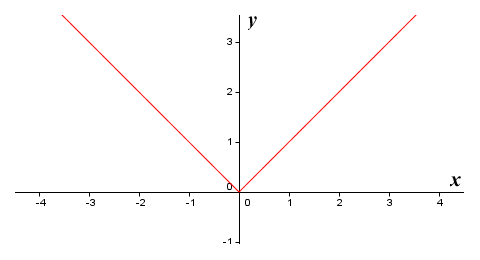The function ƒ(x) = |x| also has a single critical point

For the function ƒ(x) = |x|, the graph also has two branches. This time, however, although the branches still meet at the point x = 0, they form a corner. Once again, the function is continuous, but is not differentiable at x = 0. The derivative does not exist, because the tangents to the graph at x = 0 will have different values, depending whether they approach the point from the left or from the right.

Now consider the graph of the function ƒ(x) = 1/x: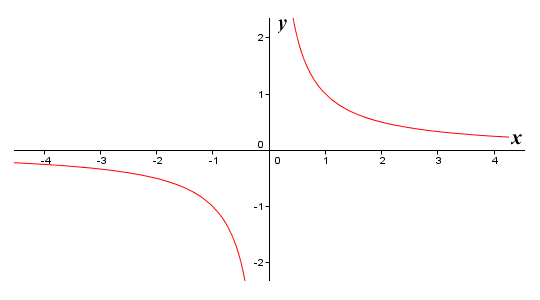The function ƒ(x) = 1/x has no critical points

The function ƒ(x) = 1/x has no critical points at all. To the right of the y axis, the value of the function approaches infinity as x approaches zero. To the left of the y axis, the value of the function approaches negative infinity as x approaches zero. The point x = 0 is not a critical point because it is not included in the domain of the function (remember that any fractional term with zero in the denominator is said to be undefined). Since the function has no critical points, it can have no local or global extrema.

Another interesting case is the graph of the function ƒ(x) = x 3:The function ƒ(x) = x 3 has a single critical point but no extrema

The function ƒ(x) = x 3 has a single critical point at x = 0, because the derivative at this point is zero. The point is clearly not a global extremum. It also fails to satisfy the criteria required for it to be a local extremum or a turning point. To the right of the y axis, the value of the function approaches infinity as x approaches infinity. To the left of the y axis, the value of the function approaches negative infinity as x approaches infinity. There are no local or global extrema.

As we have seen, a function may have zero, one, or multiple critical points. In most situations, we are interested in a function whose domain has been restricted to some defined interval. Let's assume that we have some function ƒ(x) defined on a finite interval (the domain of the function), and some point within the domain of the function at which x = c. The following possibilities exist:

• ƒ(c) does not exist (it's value is undefined)
• c is a critical point because ƒ(x) is not continuous at x = c
• c is a critical point because it is an end point of the defined interval
• c is a critical point because the derivative is not defined at x = c
• c is a critical point because the derivative of ƒ(c) is zero
• ƒ(c) exists, but c is not a critical point

Note that turning points and other kinds of extrema are always critical points, but not all critical points are turning points or extrema. Note also that some sources indicate that values of x for which the value of ƒ(x) is undefined should also be considered to be critical points. We take the view that if a point cannot be plotted on the graph of a function, it does not qualify as a critical point.

## Inflection points

Inflection points are points on the graph of a function where the concavity of the graph (i.e. the direction in which it is curving) changes from a positive concavity to a negative concavity, or vice versa. In fact, we saw an example of an inflection point during our discussion of critical points, when we looked at the graph of the function ƒ(x) = x 3. For this function, the concavity changes as the graph passes through the origin, so an inflection point occurs at the point on the graph where x = 0.

A curve that has positive concavity is said to be concave up. You can think of the curve as having a shape like a bowl, placed the right way up. A section of a curve with positive concavity for which y is decreasing as x increases is sometimes described as concave up, decreasing. A section of a curve with positive concavity for which y is increasing as x increases is similarly sometimes described as concave up, increasing. To illustrate the point, here is the graph of the function ƒ(x) = x 2.Positive concavity, or concave up

A curve that has negative concavity is said to be concave down. You can again think of the curve as having a shape like a bowl, but this time placed upside down. A section of a curve with negative concavity for which y is increasing as x increases is sometimes described as concave down, increasing. A section of a curve with negative concavity for which y is decreasing as x increases is similarly sometimes described as concave down, decreasing. To illustrate the point, here is the graph of the function ƒ(x) = -x 2.Negative concavity, or concave down

An inflection point is a point at which the concavity of a curve changes sign (i.e. from positive concavity to negative concavity, or vice versa). Note that the tangent to a curve, at a point where the concavity is positive (i.e. concave up), will always be below the curve. The tangent to a curve, at a point where the concavity is negative (i.e. concave down), will always be above the curve. Consequently, the tangent to a graph at an inflection point will intersect the graph at that point. To illustrate this point, here is the graph of the function ƒ(x) = -x 3 + 3x 2 - 2, which has an inflection point at x = 1. The tangent to the point x = 1 is shown, and clearly intersects the graph of the function at this point.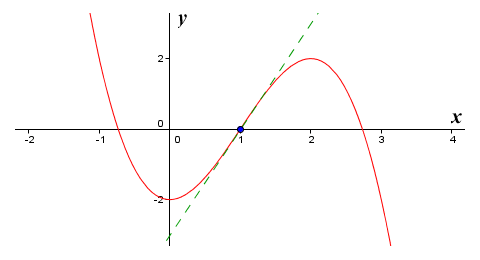The function ƒ(x) = -x 3 + 3x 2 - 2 has an inflection point at x = 1

Note that there are two distinct types of inflection point. We saw the first type when we looked at the graph of the function ƒ(x) = x 3. The derivative of this function at the inflection point is zero, which means that the inflection point is also a critical point. An inflection point at which the derivative is zero is sometimes referred to as a saddle point. The graph of the function ƒ(x) = -x 3 + 3x 2 - 2, which we have just seen, has an inflection point of the second type (sometimes called a non-stationary inflection point). The derivative at this type of inflection point is not zero, so the inflection point is not a critical point.